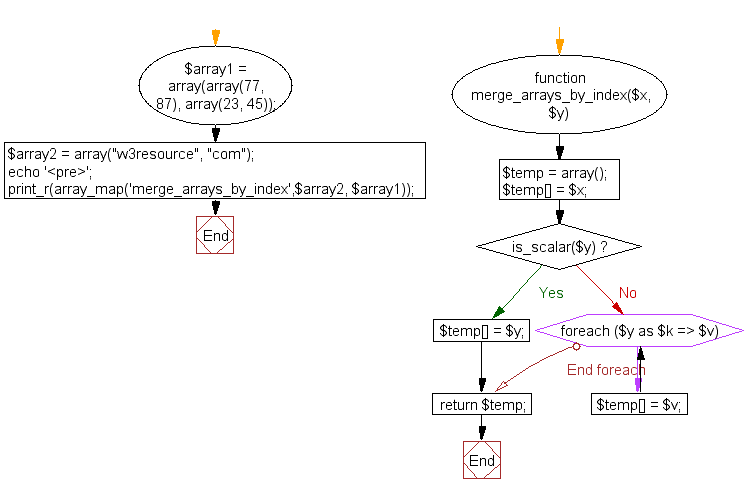﻿ PHP Array Exercise: Merge two arrays - w3resource# PHP Array Exercises : Merge two arrays

## PHP Array: Exercise-11 with Solution

Write a PHP program to merge (by index) the following two arrays.

Sample arrays :
\$array1 = array(array(77, 87), array(23, 45));
\$array2 = array("w3resource", "com");

Sample Solution:

PHP Code:

``````<?php
\$array1 = array(array(77, 87), array(23, 45));
\$array2 = array("w3resource", "com");
function merge_arrays_by_index(\$x, \$y)
{
\$temp = array(); \$temp[] = \$x; if(is_scalar(\$y))
{
\$temp[] = \$y;
}
else
{
foreach(\$y as \$k => \$v)
{
\$temp[] = \$v;
}
}
return \$temp;
}
echo '<pre>'; print_r(array_map('merge_arrays_by_index',\$array2, \$array1));
?>
```
```

Sample Output:

```<pre>Array
(
 => Array
(
 => w3resource
 => 77
 => 87
)

 => Array
(
 => com
 => 23
 => 45
)

)
```

Flowchart:PHP Code Editor:

What is the difficulty level of this exercise?

Test your Programming skills with w3resource's quiz.

﻿

## PHP: Tips of the Day

The PHP execution operator consists of backticks (' ') and is used to run shell commands. The output of the command will be returned, and may, therefore, be stored in a variable.

Example:

```// List files
\$output = 'ls';
echo "<pre>\$output</pre>";
```

Note that the execute operator and shell_exec() will give the same result.